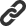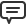### Blog Options

#### Archive

<< November 2019 >>

# Blog

Most Recent Post  |  Index List

#Introducing Sergey Gladkiy and TMS Analytics & Physics Pack

Bookmarks:

#### Monday, November 07, 2016

It's always a privilege and honour to be able to work with very smart people who are not only expert in Delphi but also expert in a specific domain. Earlier this year, Bernard Roussely and Marion Candau joined tmssoftware.com and focus so far on advanced cryptography algorithms with the highest security levels in TMS Cryptography Pack. More tools that will use this underlying strong technology are already in the pipeline. Around the same time, also Roman Yankovsky joined tmssoftware.com and the static code analysis tool TMS FixInsight is the result. With TMS FixInsight, you can bring your Delphi code to a higher quality & safer level with numerous code hints and suggestions it gives.
Today, I'm proud to announce Sergey Gladkiy joins the TMS team for working on products with a strong mathematical background. The first result is the TMS Analytics & Physics Pack. This pack offers a set of (non-visual) platform independent classes to work with mathematical expressions and units of measurements. In TMS Analytics & Physics Pack, you can do evaluation of complex multiparameter mathematical expressions, including expressions with complex numbers. You can do physical measurement conversions of all kinds. But perhaps the most amazing part is symbolic derivative calculations. In this respect, TMS Analytics & Physics Pack brings a math wizard in the form of classes that can be used in VCL and FMX apps. You can discover the power of this library via the included demo but I wanted to share some examples of symbolic derivative calculations that will boggle your mind:

Task: calculate the derivative function of:
1/(x-1)+(2*x-1)/(x+1)^2

Result:
(-(1)/(x-1)^2)+(2*(x+1)^2-(2*(x+1)^(2-1))*(2*x-1))/((x+1)^2)^2

The code to achieve this is as simple as:
```var
f,df: string;

f := '1/(x-1)+(2*x-1)/(x+1)^2';

try
if Translator.CheckSyntax(f) then
begin
df:=Translator.Derivative(f,'x');
ShowMessage('Derivative function :' + df);
end;
except
ShowMessage('Error');
end;```
Let's take another example with some trigonometric mathematical functions:

Task: calculate the derivative function of:
(sin(x)+cos(x))/x

Result:
((cos(x)+(-sin(x)))*x-(sin(x)+cos(x)))/x^2

The resulting derivative expression can then be evaluated, for example against x=2:
```var
df: string;
v: TValue;

df := '((cos(x)+(-sin(x)))*x-(sin(x)+cos(x)))/x^2';

try
if Translator.CheckSyntax(df) then
begin
v :=Translator.Calculate(df);
ShowMessage('Result :' + s:=TUtilities.SafeToString(v));
end;
except
ShowMessage('Error');
end;```
The result of this calculation is: -0,786009779256047.

I kindly invite you to explore our new TMS Analytics & Physics Pack. A fully functional trial version is available and the registered versions comes with full source code. We're eager to learn in what kind of exciting and cool applications these powerful classes will find a place!

Bruno Fierens

Bookmarks:1. Monday, November 07, 2016 at 7:06:48 PM

Hi Bruno,

Can i create a new function like Clipper IIF?

Example:

IIF( x > 0 , a + b , c * d )

Diego Diaz2. Monday, November 07, 2016 at 9:03:05 PM

Bruno Fierens3. Tuesday, November 08, 2016 at 9:23:38 PM

Can it simplify expressions? I notice that the derivative could be simplified, eg (2-1) could be reduce to (1) and in this case completely removed.

Sauro Herbert4. Wednesday, November 09, 2016 at 9:11:20 PM

''Simple'' simplifications will be done. Extended simplifications will be added in a future update.

Bruno Fierens5. Saturday, November 12, 2016 at 9:24:36 AM

From examining the documentation, am I correct in understanding that despite needing to create and use variable objects, this library only parses expression strings and doesn''t offer any symbolic manipulation capability?

Joseph Mitzen6. Saturday, November 12, 2016 at 6:06:29 PM

The main aim of the library is providing evaluation of string expressions and symbolic derivative calculation.
This library includes algorithms for symbolic expression manipulation. The simplest way is using the TExpression class - see page 23 of the PDF developers guide.

Bruno Fierens